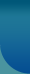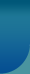Home >> Research Methods and Statistics >> Coefficient of Dispersion

## Coefficient of Dispersion

For any data, it is always desirable that the measure of dispersion is less. A small value for the measure means that the values in the data are more or less consistent, centering on their average. Suppose it is required to compare the dispersion between two or more data. For this purpose any measure of dispersion cannot be used as such for two reasons 1.the two data under study may certain to different variables such as heights and weights of the individual.

Number of marriages and ages of persons who have committed suicides etc and 2.even if the variables in the two data are the same, their averages may be different. Hence one is in need of a relative measure of dispersion which will be free from the two differences cited above. Such a measure is the coefficient of dispersion which is defined as the ratio between measure dispersion and an average. This coefficient is a constant, rendering ready comparison of dispersion between data.

There are four coefficients which are commonly referred, namely
1. Quartile coefficient of dispersion = Quartile Deviation/ Mean
2. Coefficient of variation (C.V) = Standard Deviation /Mean x 100
3. Mean Deviation/Mean
4. Mean Deviation/Median of which the first two are the most frequently used coefficients.© 2023 Sociology Guide.Com